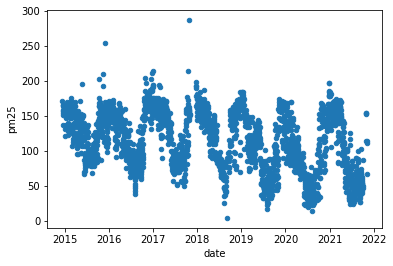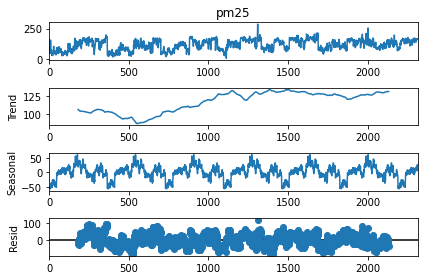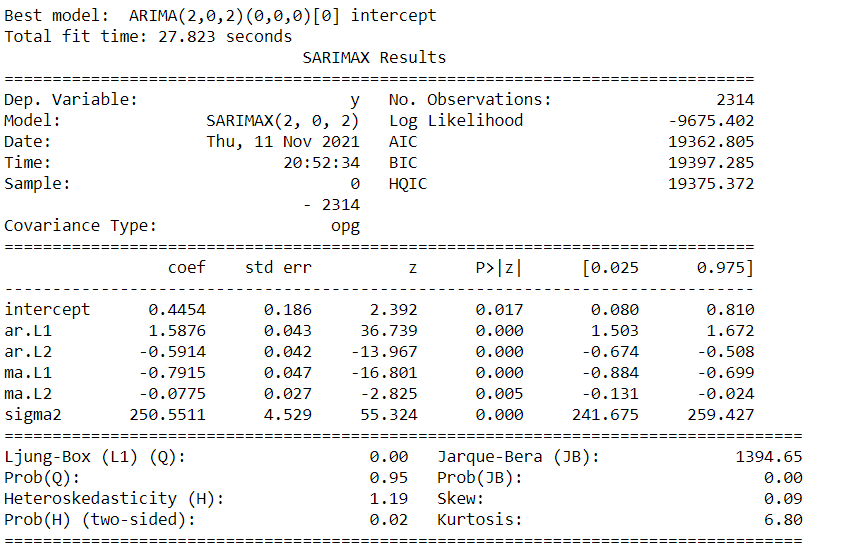# Deploying a machine learning application¶

Building a machine learning model is only half the story. Deploying this application so that the business uses it is the other half. Generally, deployment is not done by machine learning engineers or data scientists. Therefore, I see my peers lacking these skills, especially the data scientists from non-Computer Science backgrounds.
Although python developers do the deployment, data scientists need to know the basics of deploying a machine learning solution.
In the below example, I am using data taken on the amount of PM25 pollutant near my house (in Hyderabad, India) from aqicn.org. In the previous blog, I demonstrated a simple ARIMA model that can predict PM25 and discussed different ways. I want to implement this model as an API so that any website can access it for predictions. I have used pythonanywhere to deploy a flask application mentioned above.

First let me build a machine learning model. Historical data has been taken from AQICN's api

``````import pandas as pd
import matplotlib.pyplot as plt
import seaborn as sns
``````
``````data.columns = ['date', 'pm25']
data
``````
date pm25
0 2021-11-01 155
1 2021-11-02 115
2 2021-11-03 67
3 2021-11-04 112
4 2021-11-05 115
... ... ...
2309 2014-12-24 165
2310 2014-12-25 165
2311 2014-12-26 163
2312 2014-12-27 165
2313 2014-12-28 160

2314 rows × 2 columns

``````data.plot.scatter(x = 'date', y = 'pm25')
````````````from statsmodels.tsa.seasonal import seasonal_decompose
result = seasonal_decompose(data.pm25, model='additive', period = 365)
print(result.plot())
``````We can see the seasonality in the data where the pollution increases during winter and is lower during the summer months. The complete ARIMA model is discussed in a different blog post. The final results of the model are shown below:## Deployment¶

The best way to deploy the machine learning model (according to me) is to encapsulate the training and prediction logic behind the data science model along with the final model in an object. This can be done using a class as shown below. This object can be serialised/deserialised, and we need not re-write the prediction logic on the server-side every time we change the machine learning model or code. We can only change the final model file, and the application should work seamlessly. We are effectively removing the machine learning from the server-side code and instead encapsulating it on an object.
Consider the below code, which encapsulates the machine learning model:

``````import dill # dill is an alternative to pickle which is better for serialising objects along with their class definitions

class predict_pm25:
def __init__(self):
self.model = None
self.version = 1
def predict(self, date):
# This predict function can have anything
import requests
import pandas as pd
import numpy as np
from math import sqrt
import datetime
from dateutil.relativedelta import relativedelta

# Getting the actual and predictions of the last two days for ARIMA(2,0,2)
date = (datetime.datetime.strptime(date, "%Y-%m-%d")- relativedelta(days=2)).strftime("%Y-%m-%d")
response = requests.get("https://hydpm25.herokuapp.com/get_last_n_days_data", params={'date': date, 'n':2})
df = pd.DataFrame(response.json()['result'])

# Calculating the MA values
df['ma'] = df.actual - df.predicted

# Making the next prediction with ARIMA(2,0,2) model parameters shown above
df['ma_slope'] = [-0.7915, -0.0775]
df['ar_slope'] = [1.5876, -0.5914]
pred = 0.4454+sum(df.ma*df.ma_slope+df.actual*df.ar_slope) + abs(np.random.normal(0, sqrt(250.55), 1))

return pred
def save_model(self):
dill.dump(self, pkl_file)
``````

Running the code to save the model as a serialised file.

``````predict_pm = predict_pm25()
predict_pm.save_model()
``````

Flask server can be used to deploy this model. First, we set up flask server over local host. First, write the following code in a file named flask_app.py (any name except flask.py)

``````# File flask_app.py
import pandas as pd
from mc_predict import predict as machine_learning_predict # has code for the predict function

@app.route("/") # specifying the app route over the web
def base_website(): # what should happen at this route
return "Welcome to machine learning model APIs!"

@app.route('/predict', methods=['GET']) # Get request defined
def predict_request(): # what should happen at this get request
json_ = request.json
query_df = pd.DataFrame(json_)
prediction = machine_learning_predict(query_df) # we call the predict function for the machine learning model
return jsonify({'prediction': list(prediction)})

if __name__ == '__main__':
app.run(debug=True)
``````

The predict function is defined in a different file called mc_predict.py. In this function, we load (unserialise) the saved model and call the predict function in the model. Here we can observe that this is a function on the server, and it does not contain any machine learning logic. All the machine learning logic is present in the object, and changing the object can change the machine learning logic without changing this code.

``````# File mc_predict.py
import dill
def predict(date = '2021-11-12'):
model = dill.load(pkl_file) # unserialise the model
return model.predict(date)
``````

For example, the prediction for '2021-11-12' is

``````predict()
``````
``````array([142.32741472])
``````

That's it. We have our local deployment ready. We will have to go to the folder where these files are present and type 'python flask_app.py'. We will get the app running on http://127.0.0.1:5000/.